# Evaluation of the direct and diffused component of solar radiation starting from global radiation measurements: Preliminary analysis

Evaluation of the direct and diffused component of solar radiation starting from global radiation measurements: Preliminary analysis

Concettina MarinoAntonino Nucara Matilde Pietrafesa

Department of Civil, Energy, Environmental and Material Engineering (DICEAM), “Mediterranea” University of Reggio Calabria, Reggio Calabria (89124), Italy

Corresponding Author Email:
concettina.marino@unirc.it
Page:
317-322
|
DOI:
https://doi.org/10.18280/mmep.050407
7 July 2018
|
Accepted:
14 November 2018
|
Published:
31 December 2018
| Citation

OPEN ACCESS

Abstract:

This article aims to devise an analytical methodology suitable for assessing the various components of the solar radiation (direct, diffused by the sky vault, reflected by the ground and other surrounding surfaces). As input, it uses measured data of the global solar irradiance coming from six perpendicular space directions (South, North, Est, West and the two vertical directions). Assessing the contribute of the component of solar radiation which is reflected by the surrounding surfaces on a horizontal plane, the proposed method is suited to being applied in areas (e.g. urban settings) where an optimal installation of the measurement apparatus (devoid of objects reflecting solar radiation) is not possible. Furthermore, it allows the actual sky view factor to be taken into account, so that the influence of objects or surfaces, partially shading the view of the sky is evaluated. In addition, the experimental apparatus generally used for the assessment of the mean radiant temperature in outdoor environment may be exploited, thus allowing a more comprehensive control of the climate conditions of the analysed site.

Keywords:

solar radiation, direct-diffuse and reflected component, six-directional technique

1. Introduction

Urban planning is currently considered a crucial factor within the actions aimed at mitigating the effect and the consequences of global warming. It involves different fields of intervention [1-2], which also regard the analysis and control of the outdoor climatic conditions [3-4].

As a matter of fact, local weather conditions in urban areas affect the energy consumption of buildings , the chance of renewable source exploitation  and determine the quality of outdoor urban space, thus influencing various human behaviors  and related activities as pedestrian traffic and modal choices of the transportation systems, whose rationalization is one of the crucial objective of the action plans adopted by local authorities in view of tackling mitigation of climate change .

Solar radiation levels are the main features characterizing the weather conditions of a site  and influence the real effectiveness of the actions usually designed to foster a sustainable development of urban settlements. Actually, they affect the chances of passive lighting , renewable source energy production  (either photovoltaic or thermal), thermal gains of indoor space and, hence, building energy consumption . Furthermore, they are essential input data for the assessment of the outdoor comfort conditions  and, therefore, for the evaluation of the actual suitability of outdoor spaces.

Among solar radiation components usually gathered in meteorological stations observed in the world, global radiation on horizontal surface, namely the sum of the direct and diffuse components, is the most frequently measured quantity .

That circumstance is essentially due to the maintenance requirements which characterize the instrumental apparatus needed for measurements of direct and diffuse components (pyranometers equipped with either solar tracking systems or shadow band mobile structures).

Nevertheless, the knowledge of both the two components is a crucial issue for the analysis which the urban planning process relies on. As an instance, passive lighting mostly depends on diffuse radiation, whereas photovoltaic conversion is essentially determined by direct solar radiation.

In this context, this article aims to devise an analytical methodology for the assessment of the various components of the solar radiation (direct, diffused by the sky vault and reflected by the ground and other surrounding surfaces), using measured data of global irradiance coming from six perpendicular space directions (South, North, Est, West and the two vertical directions) as input.

Assessing the contribute of the component of solar radiation which is reflected by the surrounding surfaces on a horizontal plane, the proposed method is suited to being applied in areas (e.g. urban settings) where an optimal installation of the measurement apparatus (devoid of objects reflecting solar radiation) is not possible.

Furthermore, it allows the actual sky view factor to be taken into account, so that the resulted diffuse radiation term accounting for the presence of objects or surfaces partially shading the view of the sky, is evaluated.

In addition, the experimental apparatus generally used for the assessment of the mean radiant temperature in outdoor environment may be exploited  (six-dimensional technique), thus using the same equipment to obtain a more comprehensive control of the climate conditions of the analysed area.

Finally, in order to carry out a preliminary test regarding the reliability of the procedure, its output was compared to the outcome of the Diffuse Fraction Correlation Model, reported in .

2. Experimental Apparatus

The instrument setup used for the radiant flux measurement is shown in Figure 1.

## figure_1.pngFigure 1. Experimental apparatus

It consists of three probes installed on a pole. Each probe is a CNR4TM net radiometer (Kipp and Zonen), which, in turn, consists of a pyranometer pair, disposed on the opposite surfaces of the probe’s plane, and a pyrgeometer pair in a similar conﬁguration. The pyranometer pair measures the short-wave radiation, whereas the pyrgeometer pair measures long-wave radiation.

The measured data are collected through a set of two loggers (QML102TM, VAISALA) which are connected to a laptop, located in a nearby container, through a Ethernet port.

The measure point (P, Figure 2) is located inside the courtyard of the Mediterranean University on an area which is equipped with all the needed networks.

## figure_2.pngFigure 2. Mediterranean University campus - Measurement point P

3. Methodology

The proposed method aims to the assessment of the direct and diffuse components of solar radiation in urban environments, where an optimal placement of the measure apparatus, devoid of interfering obstacles and reflective surfaces, is often not possible owing to the morphology of the available areas.

The needed input data are the values of the solar irradiance coming from six perpendicular space directions, measured by means of six pyranometers.

If a spatial reference system, x, y, z, is used, the six solar irradiance variables may be denoted as follows:

Ix+solar irradiance measured by the sensor facing the positive direction of the x axis;

Ix- solar irradiance measured by the sensor facing the negative direction of the x axis;

Iy+solar irradiance measured by the sensor facing the positive direction of the y axis;

Iy- solar irradiance measured by the sensor facing the negative direction of the y axis;

Iz+solar irradiance measured by the sensor facing the positive direction of the z axis (upward);

Iz- solar irradiance measured by the sensor facing the negative direction of the z axis (downward);

Within these conditions, considering that the solar radiation received by a plane on the ground surface consists of three component (the direct radiation coming from the solar disk, the diffuse radiation derived from the sky vault, and the reflected radiation coming from surrounding surfaces) the following equation system can be provided:

$\left\{\begin{array}{c}{R_{b x^{+}} I_{b h}+I_{d, r x}+=I_{x^{+}}} \\ {R_{b x^{-}} I_{b h}+I_{d, r x^{-}}=I_{x^{-}}} \\ {R_{b y^{+}} I_{b h}+I_{d, r y^{+}}=I_{y^{+}}} \\ {R_{b y^{-}} I_{b h}+I_{d, r y^{+}}=I_{y^{-}}} \\ {I_{b h}+I_{d, r z^{+}}=I_{z^{+}}}\end{array}\right.$  (1)

where: $I_{b h}$ is the direct component of solar radiation on the horizontal plane; $I_{d, r}$ is the sum of the diffuse and reflected component of solar radiation on each surface facing each of the six directions (x+, x-, y+, y-, z+, z-).

Rb indicates the conversion factor for the direct solar irradiance on the involved surface. It depends on the incidence angle $\theta$ (namely the angle between the solar beam and the straight line normal to the surface) and on the solar altitude $\alpha$:

$R_{b}=\frac{\cos \theta}{\operatorname{sen} \alpha}$  (2)

The equation systems (1) consists of 5 equations, but the unknown variables are six: $I_{b h}, I_{d, r x^{+}}, I_{d, r x^{-}}, I_{d, r y^{+}}, I_{d, r y^{-}}, I_{d, r z^{+}}$.

The sixth equation is obtained considering the specific effect of the reflections from the surrounding surfaces and of the radiation diffused from the sky vault, which does not depend on the orientation of the struck plane and hence is constant for all the vertical surface. Specifically, the following balance can be written:

$I_{d, r x^{+}}+I_{d, r x^{-}}+I_{d, r y^{+}}+I_{d, r y^{-}}-4 \frac{I_{z}^{-}}{2}=\Delta$  (3)

where $\frac{\mathrm{I}_{\mathrm{Z}^{-}}}{2}$ is the radiation reflected by the ground on the vertical plane.

Regarding the term $\Delta$, it was introduced to take two possible occurrences into account: if no other reflecting surface but the ground is present within the surrounding environment, $\Delta$ is equal to overall radiation diffused from the sky and globally shining on the four vertical planes (x+, x-, y+, y-); on the contrary, if reflections from the surrounding environment (other than the ground) cannot be neglected, they are included in the term $\Delta$.

Its value can be assessed whether the following conditions are laid down:

$\left\{\begin{array}{c}{\Delta>0} \\ {I_{b h} \geq 0} \\ {I_{d, r x^{+}} \geq \frac{I_{z}^{-}}{2}+0.05 \times\left(I_{b h}+I_{d, r z^{+}}\right)} \\ {I_{d, r x^{-}} \geq \frac{I_{z}^{-}}{2}+0.05 \times\left(I_{b h}+I_{d, r z^{+}}\right)} \\ {I_{d, r y^{+}} \geq \frac{I_{z}^{-}}{2}+0.05 \times\left(I_{b h}+I_{d, r z^{+}}\right)} \\ {I_{d, r y^{+}} \geq \frac{I_{z^{-}}}{2}+0.05 \times\left(I_{b h}+I_{d, r z^{+}}\right)} \\ {I_{d, r z^{+}} \geq 0.10 \times\left(I_{b h}+I_{d, r z^{+}}\right)}\end{array}\right.$  (4)

In short, combining eq. (1), eq. (3), a new system is obtained:

$\left\{\begin{array}{c} R_{b x}+I_{b h}+I_{d, r x^{+}}=I_{x^{+}} \\ R_{b x^{-}} I_{b h}+I_{d, r x^{-}}=I_{x^{-}} \\ R_{b y}+I_{b h}+I_{d, r y^{+}}=I_{y^{+}} \\ R_{b y^{-}}-I_{b h}+I_{d, r y^{-}}=I_{y^{-}}\\ I_{b h}+I_{d, r z^{+}}=I_{z^{+}} \\ I_{d, r x^{+}}+I_{d, r x^{-}}+I_{d, r y^{+}}+I_{d, r y^{-}}=2 I_{z^{-}}+\Delta \end{array}\right.$ (5)

which must be solved under the conditions expressed by eqs. (4)

Its solution allows the assessment of the unknown variables $I_{b h}, I_{d, r x^{+}}, I_{d, r x^{-}}, I_{d, r y^{+}}, I_{d, r y^{-}}, I_{d, r z^{+}}$ and the term $\Delta$.

In order to assess the diffuse and reflected components on the horizontal plane, whose sum is equal to $I_{d, r z^{+}}$, a further step is needed.

Firstly eq. (3) can be also written as:

$4 \frac{I_{d h}}{2}+I_{r x^{+}}+I_{r x^{-}}+I_{r y^{+}}+I_{r y^{-}}-4 \frac{I_{z}^{-}}{2}=\Delta$  (6)

where $I_{d h}$ is the radiation diffused by the sky vault on the horizontal plane; $I_{r x^{+}}, I_{r x^{-}}, I_{r y^{+}}, I_{r y^{-}}$ are the portions of solar radiation reflected by the surrounding surfaces, other than the ground, on the four vertical planes.

If a new variable $\mathrm{I}_{\mathrm{r}}$ is defined as:

$I_{r}=I_{r x^{+}}+I_{r x^{-}}+I_{r y^{+}}+I_{r y^{-}}$    (7)

thus Eq. (5) can be written as:

$2 I_{d h}+I_{r}=2 I_{z^{-}}+\Delta$ (8)

So that, following a procedure similar to the one formerly described, the unknown variables $I_{d h}$ and $\mathrm{I}_{\mathrm{r}}$ can be assessed by solving the system:

$\left\{\begin{array}{c}{2 I_{d h}+I_{r}=2 I_{z}^-+\Delta} \\ {I_{d h}+I_{r z}^+=I_{d, r z^{+}}} {I_{r}=2 I_z^-+\Delta_{1}} \\ {I_{d h} \geq 0.10 \times\left(I_{b h}+I_{d h}\right)} \\ {I_{r} \geq 0} \\ {0 \leq I_{r z}^+<0.50 \times I_{d h}}\end{array}\right.$  (9)

where the unknown variables are: $I_{d h}, I_{r}, I_{r z}^+$ and $I_{r Z}^+$ is the component of solar radiation reflected by the surrounding surfaces on the horizontal plane.

Solving equation systems (5), within the conditions of eqs. (4), and (9) consecutively, all the needed unknown variables are assessed.

4. Case Study

Data gathered during four days characterized by different sky conditions (clear, partially cloudy and cloudy) were used to examine the procedure accountability.

Specifically, the four considered days are: 21/06/2017, 21/09/2017, 21/12/2017 and 21/03/2018.

Furthermore, in order to carry out a preliminary test regarding the reliability of the procedure, its output was compared to the outcome of the Diffuse Fraction Correlation Model, reported in  and used to assess the direct and diffuse components of solar radiation from global data. Specifically:

$\frac{I_{d h}}{I_{b h}+I_{d h}}=\left\{\begin{array}{ccc}{1.020-0.248 k_{t}} & {\text { if }} & {0.0 \leq k_{t} \leq 0.3} \\ {1.450-1.670} {k_{t}} & {\text { if }} & {0.3<k_{t}<0.78} \\ {0.147} & {\text { if }} & {0.78 \leq k_{t}}\end{array}\right.$ (10)

where kt is the ratio of global horizontal to extraterrestrial radiation and $\left(I_{b h}+I_{d h}\right)$  is the measured global solar radiation on the horizontal plane.

4.1 Input data

## figure_3.pngFigure 3. Solar irradiance 21/06/2017- hourly data

## figure_4.png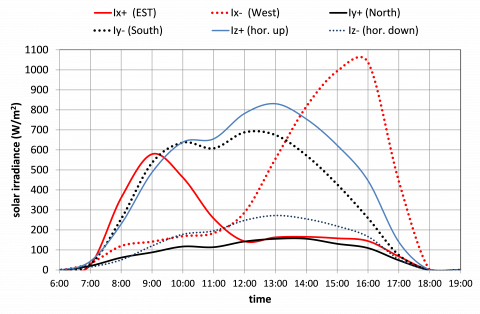Figure 4. Solar irradiance 21/09/2017- hourly data

## figure_5.pngFigure 5. Solar irradiance 21/12/2017- hourly data

## figure_6.png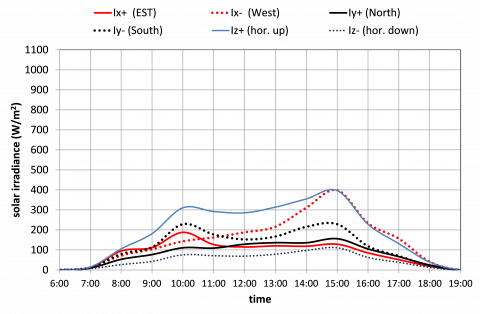Figure 6. Solar irradiance 21/03/2018- hourly data

Figure 3, Figure 4, Figure 5 and Figure 6 depict the measured data used as input. They are the hourly average values of the solar irradiances coming from the six spatial directions, respectively pointing South, North, Est, West, up and down.

It is worth noting the different levels of solar irradiance characterizing the four selected days, respectively corresponding to different sky conditions.

5. Result and Discussion

Comparisons among the results of the proposed procedure and of the Diffuse Fraction Correlation Model are reported in Figure 7, Figure 8, Figure 9 and Figure 10.

## figure_7.png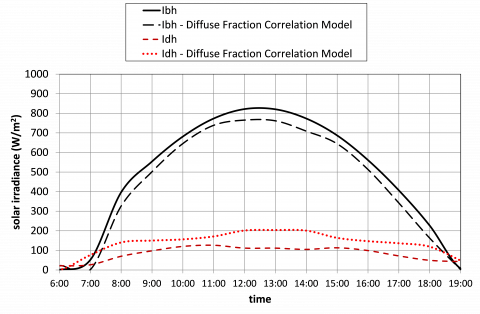Figure 7. Solar radiation: comparison among the results of the proposed method and the outcome of the Diffuse fraction correlation model- 21/06/2017- hourly data

## figure_8.pngFigure 8. Solar radiation: comparison among the results of the proposed method and the outcome of the Diffuse fraction correlation model- 21/09/2017- hourly data

## figure_9.png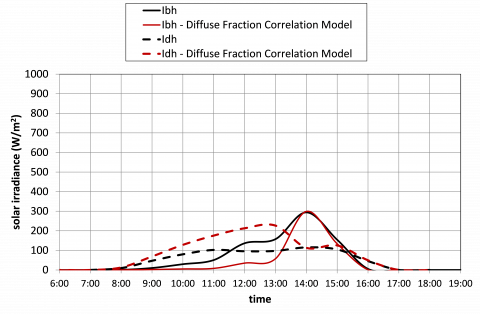Figure 9. Solar radiation: comparison among the results of the proposed method and the outcome of the Diffuse fraction correlation model- 21/12/2017- hourly data

All the components of solar radiation there reported (direct $I_{bh}$, and diffuse $I_{dh}$) shine on the horizontal plane.

Firstly, it is worth noting that the two methods yield results which show similar time trends. Therefore, the proposed procedure seems quite reliable from this point of view.

Furthermore, it estimates values of the direct component of solar radiation which tend to be higher than the ones calculated by means of the Diffuse Fraction Correlation Model. Conversely, the opposite circumstance regards the diffuse component: the values calculated by means of the proposed procedure are, by and large, lower.

## figure_10.png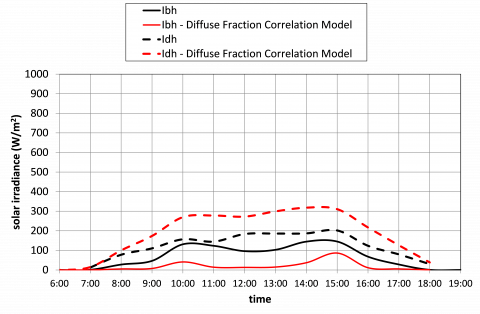Figure 10. Solar radiation: comparison among the results of the proposed method and the outcome of the Diffuse fraction correlation model- 21/03/2018- hourly data

This occurrence is presumably due to the configuration of the site where the measures were performed, namely to the presence of a sky view factor which is actually different from 1.

The proposed procedure is able to take this circumstance into account, thus yielding the actual value of the radiation diffused from the visible sky vault.

The effect of the presence of reflective surfaces is deducible from Figure 11, which reports the radiation, $I_{ir}^+$ , they reflect on the horizontal plane.

## figure_11.pngFigure 11. Solar radiation component reflected on the horizontal plane by the surfaces located nearby the measure point

6. Conclusions

This article aims to devise an analytical methodology for the assessment of the various components of the solar radiation (direct, diffused by the sky vault and reflected by the ground and other surrounding surfaces), using measured data of global irradiance coming from six perpendicular space directions (South, North, Est, West and the two vertical directions) as input.

It is suited to being applied in areas (e.g. urban settings) where an optimal installation of the measurement apparatus (devoid of objects reflecting solar radiation) is not possible.

As a matter of fact, it allows the actual sky view factor to be taken into account, and the contribute of the component of solar radiation which is reflected by the surrounding surfaces on a horizontal plane to be calculated.

Preliminary analysis of the results of the proposed method are encouraging: on balance, comparison to the outcome of a well-known statistical model seems to yield a positive response.

However, further analysis regarding both the validity limits of the proposed model and the consistency of the results are needed for a definitive judgment on its reliability.

An experimental campaign is being planned in order to fulfil this task.

Acknowledgment

This work was carried out within the research project n. 201594LT3F, “La ricerca per i PAES: una piattaforma per le municipalità partecipanti al Patto dei Sindaci (Research for SEAP: a platform for municipalities taking part in the Covenant of Mayors)”, which is funded by the PRIN (Programmi di Ricerca Scientifica di Rilevante Interesse Nazionale) of the Italian Ministry of Education, University and Research.

Nomenclature
References

 Margolin V. (2015). The good city: Design for sustainability. She Ji: The Journal of Design, Economics, and Innovation 1(1): 34–43. http://doi.org/10.1016/J.SHEJI.2015.07.001

 Leyzerova A, Sharovarova E, Alekhin V. (2016). Sustainable strategies of urban planning. Procedia Eng. 150: 2055-2061. http://doi.org/10.1016/j.proeng.2016.07.299

 Axhausen KW, Batty M, Bruse M, Flourentzou F, Haldi F, Kämpf J, Perez D, Rasheed A, Robinson D. (2011). Computer modelling for sustainable urban design. Routledge. Taylor & Francis.

 Nikolopoulou M, Lykoudis S. (2007). Use of outdoor spaces and microclimate in a Mediterranean urban area. Build. Environ. 42: 3691-3707. http://doi.org/10.1016/j.buildenv.2006.09.008.

 Cannistraro G, Giaconia C, Pietrafesa M, Rizzo G. (1995). Reduced weather data for building climatization and application to 29 European locations. Energy 20(7): 637–646. http://doi.org/10.1016/0360-5442(94)00100-H

 La Gennusa M, Lascari G, Rizzo G, Scaccianoce G, Sorrentino G. (2011). A model for predicting the potential diffusion of solar energy systems in complex urban environments. Energy Policy 39: 5335-5343. http://doi.org/10.1016/j.enpol.2011.05.031

 Marino C, Nucara A, Peri G, Pietrafesa M, Pudano A, Rizzo G. (2015). An MAS-based subjective model for indoor adaptive thermal comfort. Sci. Technol. Built Environ. 21: 114-125. http://doi.org/10.1080/10789669.2014.980683

 Chen L, Ng E. (2012). Outdoor thermal comfort and outdoor activities: A review of research in the past decade. Cities 29: 118-125. http://doi.org/10.1016/j.cities.2011.08.006

 Oteiza P, Pérez-Burgos A. (2012). Diffuse illuminance availability on horizontal and vertical surfaces at Madrid. Spain. Energy Convers. Manag. 64: 313-319. http://doi.org/10.1016/j.enconman.2012.05.022

 Chantana J, Ueno S, Ota Y, Nishioka K, Minemoto T, Uniqueness verification of direct solar spectral index for estimating outdoor performance of concentrator photovoltaic systems. Renew. Energy 75: 762-766. http://doi.org/10.1016/j.renene.2014.10.059

 Marino C, Nucara A, Pietrafesa M. (2017). Does window-to-wall ratio have a significant effect on the energy consumption of buildings? A parametric analysis in Italian climate conditions. J. Build. Eng. 13: 169-183. http://doi.org/10.1016/j.jobe.2017.08.001

 Dal Pai A, Escobedo JF, Dal Pai E, de Oliveira AP, Soares JR, Codato G. (2016). MEO shadowring method for measuring diffuse solar irradiance: Corrections based on sky cover, renew. Energy 99: 754-763. http://doi.org/10.1016/j.renene.2016.07.026

 Höppe P. (1992). A new procedure to determine the mean radiant temperature outdoors. Wetter Und Leb. 44: 147-151.

 Reindl DT, Beckman WA, Duﬃe JA. (1990). Diﬀuse fraction correlations. Sol. Energy 45: 1-7. http://doi.org/10.1016/0038-092X(90)90060-P